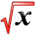# Second Order Linear Equations With Constant CoefficientThis article is part of the MathHelp Tutoring Wiki

The equation is like this:

1*. a*y' '(t)+b*y'(t)+c*y(t)=0 and a,b,c are constants a=++++0 Our goal is to find y(t)

Step 1:

Look for a solution in the form

2*. y(t)= e^pt (p is a real number to be determined)

Plug 2* into 1*

y'(t)= e^pt *(pt)'

y'(t)=p*e^pt

y(t)=p^2*e^pt

a*(p^2)*(e^pt)+b*p*e^pt+c*e^pt=0

e^pt(ap^2+bp+c)=0

a*p^2+b*p+c=0

delta=b^2-4ac

Theorem:

(a) if delta>0

y(t)= c1*e^(p1*t)+c2*e^(p2*t)

p1,p2=-b+/- squr(delta)

c1 and c2 are constants of integration

(b) if delta=0,

p1,p2=-b/2a

y(t)=C1*e^p1t+C2*t*e^p2t